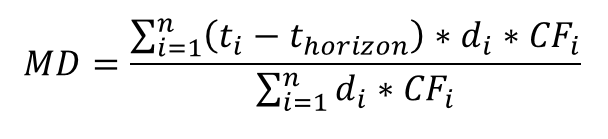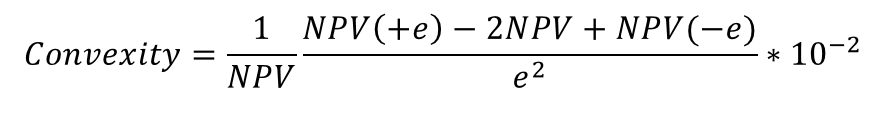# SAP TRM 模块提供的敏感度指标Sensitivity Calculation.xlsx

• Macaulay duration

Macaulay duration is not calculated for options and FX, since this is not relevant from a business perspective.

• Macaulay      Duration of a Fixed-Rate Transaction

The Macaulay duration of a fixed-rate transaction is the quotient of the total of the cash flows weighted at the points in time of the payments, and the NPV of the transaction on the horizon.where CF i   are the cash flows of the transaction at time point t i , t horizon   is the horizon date, and d i   the discount factors of time point t i   for horizon date t horizon .

• basis point      sensitivity ( Price Value of Basis Point, PVBP or as BPV for Bond)

The basis point value describes the change in the market value in the event of an increase in market interest rates for all terms, each by one basis point (0.01%). It specifies absolute changes.

PVBP = NPV(+e) - NPV

• Fisher-Weil Duration

Fisher-Weil duration describes the elasticity of the NPV to interest rate changes.

The system calculates the Fisher-Weil duration as the difference quotient of the NPVs by shifting the underlying market rates:where NPV is the net present value of the transaction, and NPV(+ e ) and NPV(- e ) is the NPV of the transaction after the market rates have been shifted upwards or downwards. A basis point is used as the value of the shift (= 0.01% = 0.0001). The system calculates the Fisher-Weil duration in years.

If the  Continuous Compounding  indicator is set, then the system uses the constant interest calculation method, regardless of the term of the cash flow:

MD = term

FWD = term = MD

• Convexity

The convexity is the sensitivity of the NPV to changes in the interest rate described by the curvature of the price curve.

The system calculates the convexity as the difference quotients by shifting the underlying market interest rates:where NPV is the net present value of the transaction, and NPV(+ e ) and NPV(- e ) is the NPV of the transaction after the market rates have been shifted upwards or downwards. A basis point is used as the value of the shift (= 0.01% = 0.0001). The factor 10 -2   is the standardization.

The convexity of a portfolio is the mean value of the convexities calculated for the individual transactions and weighted by their NPVs.

• Yield to Maturity YTM

The Yield to Maturity (YTM) is the interest rate at which the net present value of the future cash flows is equal to the actual market value of the investment.

The system calculates the YTM by solving the equation specified below:

• CF i   is the fixed future cash flow of the investment at the time      point t i
• MV(t horizon ) is the market value of the      investment at horizon date
• Modified Duration (MODDUR)

Modified duration is an adjusted measure of the duration of an investment, in which Macaulay's duration value is adjusted with the yield to maturity (YTM) of the investment.

For      fixed-rate/variable-rate transactions other than a swap, the system      calculates the modified duration as follows:

MODDUR = MD / (1 + YTM)

SAP 金融风险管理产品专家

• 博文量
211
• 访问量
534340